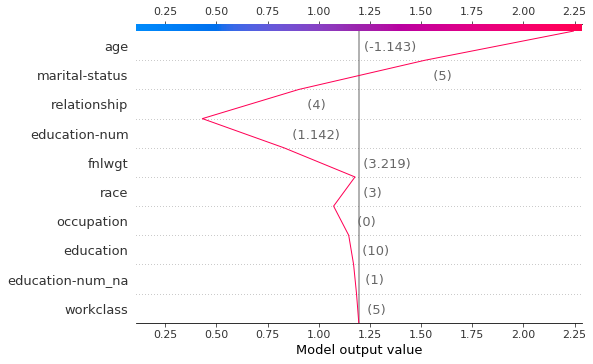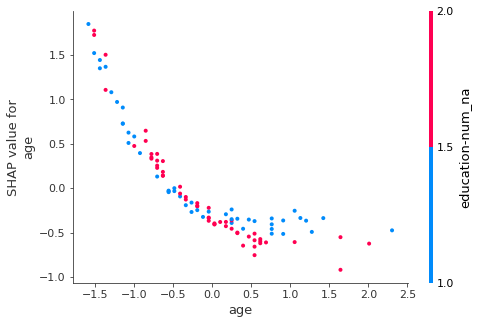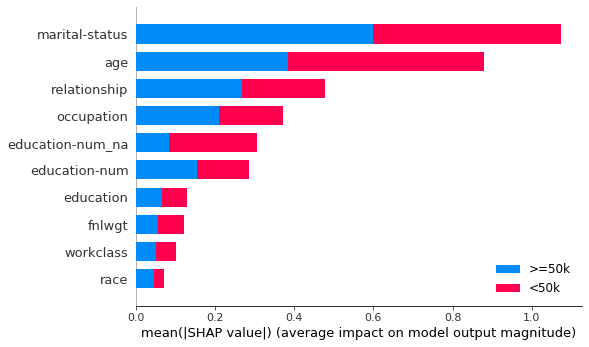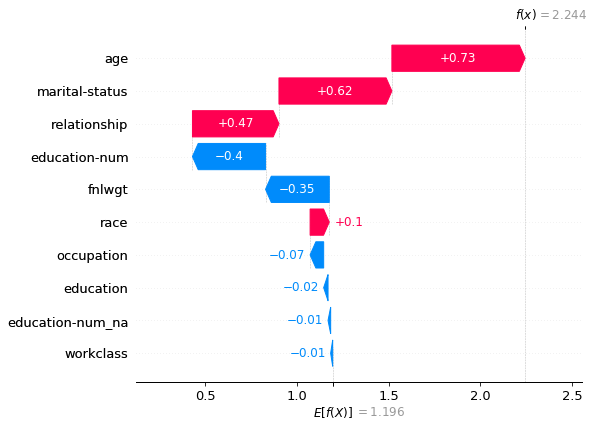This module contains the base for SHAP interpretation.

First let's train a model to analyze

path = untar_data(URLs.ADULT_SAMPLE)

dep_var = 'salary'
cat_names = ['workclass', 'education', 'marital-status', 'occupation', 'relationship', 'race']
cont_names = ['age', 'fnlwgt', 'education-num']
procs = [Categorify, FillMissing, Normalize]

splits = IndexSplitter(list(range(800,1000)))(range_of(df))
to = TabularPandas(df, procs, cat_names, cont_names, y_names="salary", splits=splits)


And fit it

learn = tabular_learner(dls, layers=[200,100], metrics=accuracy)
learn.fit(1, 1e-2)

epoch train_loss valid_loss accuracy time
0 0.371466 0.418326 0.815000 00:04

## classShapInterpretation[source]

ShapInterpretation(learn:TabularLearner, test_data=None, link='identity', l1_reg='auto', n_samples=128, **kwargs)

Base interpereter to use the SHAP interpretation library

This class allows you to utilize various methods within the SHAP interpretation library. Currently summary_plot, dependence_plot, waterfall_plot, force_plot, and decision_plot are supported.

• test_data should be either a Pandas dataframe or a TabularDataLoader. If not, 100 random rows of the training data will be used instead.
• link can either be "identity" or "logit". A generalized linear model link to connect the feature importance values to the model output. Since the feature importance values, phi, sum up to the model output, it often makes sense to connect them to the ouput with a link function where link(outout) = sum(phi). If the model output is a probability then the LogitLink link function makes the feature importance values have log-odds units.
• n_samples can either be "auto" or an integer value. This is the number of times to re-evaluate the model when explaining each predictions. More samples leads to lower variance estimations of the SHAP values
• l1_reg can be:an integer value representing the number of features, "auto", "aic", "bic", or a float value. The l1 regularization to use for feature selection (the estimation procedure is based on a debiased lasso). The auto option currently uses "aic" when less that 20% of the possible sample space is enumerated, otherwise it uses no regularization.
exp = ShapInterpretation(learn)


exp = ShapInterpretation(learn, df.iloc[:2])


exp = ShapInterpretation(learn, learn.dls.test_dl(df.iloc[:100]))


### decision_plot

Visualizes a model's decisions using cumulative SHAP values. Accepts a class_id which is used to indicate the class of interest for a classification model. It can either be an int or str representation for a class of choice. Each colored line in the plot represents the model's prediction for a single observation. If no index is passed in to use from the data, it will default to the first ten samples on the test set. Note:plotting too many samples at once can make the plot illegible. For an up-to-date list of parameters, see here and for more information see here

exp.decision_plot(class_id=0, row_idx=10)

Classification model detected, displaying score for the class <50k.
(use class_id to specify another class)
Displaying row 10 of 100 (use row_idx to specify another row)### dependence_plot

Plots the value of a variable on the x-axis and the SHAP value of the same variable on the y-axis. Accepts a class_id and variable_name. class_id is used to indicate the class of interest for a classification model. It can either be an int or str representation for a class of choice. This plot shows how the model depends on the given variable. Vertical dispersion of the datapoints represent interaction effects. Gray ticks along the y-axis are datapoints where the variable's values were NaN. For an up-to-date list of parameters, see here and for more information see here

exp.dependence_plot('age', class_id=0)

Classification model detected, displaying score for the class <50k.
(use class_id to specify another class)### force_plot

Visualizes the SHAP values with an added force layout. Accepts a class_id which is used to indicate the class of interest for a classification model. It can either be an int or str representation for a class of choice. matplotlib determines if it should be shown using matplotlib or in JavaScript. For an up-to-date list of parameters, see here

exp.force_plot(class_id=0)

Classification model detected, displaying score for the class <50k.
(use class_id to specify another class)Visualization omitted, Javascript library not loaded!
Have you run initjs() in this notebook? If this notebook was from another user you must also trust this notebook (File -> Trust notebook). If you are viewing this notebook on github the Javascript has been stripped for security. If you are using JupyterLab this error is because a JupyterLab extension has not yet been written.

### summary_plot

Displays the SHAP values (which can be interpreted for feature importance) For an up-to-date list of parameters, see here

exp.summary_plot()### waterfall_plot

Plots an explanation of a single prediction as a waterfall plot. Accepts a row_index and class_id. row_index is the index of the row chosen in test_data to be analyzed, which defaults to zero. Accepts a class_id which is used to indicate the class of interest for a classification model. It can either be an int or str representation for a class of choice. For an up-to-date list of parameters, see here

exp.waterfall_plot(row_idx=10)

Classification model detected, displaying score for the class <50k.
(use class_id to specify another class)
Displaying row 10 of 100 (use row_idx to specify another row)